### Asymptotic approach

As above the likelihood is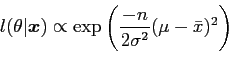(34)

so the log-likelihood is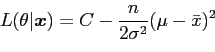(35)

where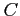is a constant. Differentiating and equating to zero gives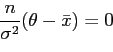(36)

so that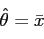(37)

and a second differentiation gives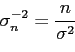(38)

giving, in this case,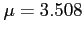and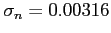.

A histogram of 1000 samples from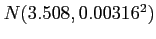is shown in figure 3. While not a good density estimator, a histogram is useful for the gross comparison of two samples needed here. Note that this histogram appears to come from a distribution with a smaller standard deviation than that shown in figure 1; the predictive sample is a safer estimate as it allows for the uncertainty in the point density estimation.danny 2009-07-23